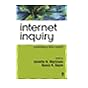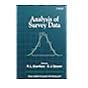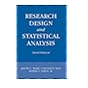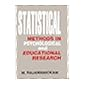Normal view MARC view ISBD view

# Research Design and Statistical Analysis by Jereome L. Myres, Arnold D. Well and Robert F. Lorch

Material type:BookPublisher: London Routledge 2010Edition: 3rd ed.Description: 809p.ISBN: 9780805864311.Subject(s): Experimental Design | Mathematical StatisticsDDC classification: 001.422
Tags from this library: No tags from this library for this title.
Item type Current location Collection Call number Status Date due Barcode Item holdsBook
General 001.422 MYE (Browse shelf) Available M0028368
Total holds: 0
##### Browsing Institute of Management Shelves , Collection code: General Close shelf browserNo cover image available 001.4202854678 MAR Internet Inquiry: Conversations about Method 001.422 ANA Analysis of Survey Data 001.422 ARG Statistics For Research: With a Guide to SPSS 001.422 MYE Research Design and Statistical Analysis 001.422 RAJ Statistical Methods in Psychological and Educational Research 001.424 PRI Introduction to Simulation and SLAM 001.424 SCH Simulation of Discrete Stochastic Systems

pt. 1 Foundations of Research Design and Data Analysis
ch. 1 Planning the Research
1.1. Overview
1.2. The Independent Variable
1.3. The Dependent Variable
1.4. The Subject Population
1.5. Nuisance Variables
1.6. Research Design
1.7. Statistical Analyses
1.8. Generalizing Conclusions
1.9. Summary
ch. 2 Exploring the Data
2.1. Overview
2.2. Plots of Data Distributions
2.3. Measures of Location and Spread
2.4. Standardized (z) Scores
2.5. Measures of the Shape of a Distribution
2.6. Comparing Two Data Sets
2.7. Relationships Among Quantitative Variables
2.8. Summary
ch. 3 Basic Concepts in Probability
3.1. Overview
3.2. Basic Concepts for Analyzing the Structure of Events
3.3. Computing Probabilities
3.4. Probability Distributions
3.5. Connecting Probability Theory to Data
3.6. Summary
ch. 4 Developing the Fundamentals of Hypothesis Testing Using the Binomial Distribution
4.1. Overview
Contents note continued: 4.2. What Do We Need to Know to Test a Hypothesis?
4.3. The Binomial Distribution
4.4. Hypothesis Testing
4.5. The Power of a Statistical Test
4.6. When Assumptions Fail
4.7. Summary
ch. 5 Further Development of the Foundations of Statistical Inference
5.1. Overview
5.2. Using Sample Statistics to Estimate Population Parameters
5.3. The Sampling Distribution of the Sample Mean
5.4. The Normal Distribution
5.6. The Power of the z Test
5.7. Validity of Assumptions
5.8. Relationships Between the Normal and Other Distributions
5.9. Summary
ch. 6 The t Distribution and its Applications
6.1. Overview
6.2. Design Considerations: Independent Groups or Correlated Scores?
6.3. The t Distribution
6.4. Data Analyses in the Independent-Groups Design
6.5. Data Analyses in the Correlated-Scores Design
6.6. Assumptions Underlying the Application of the t Distribution
Contents note continued:
6.7. Measuring the Standardized Effect Size: Cohen's d
6.8. Deciding on Sample Size
6.9. Post Hoc Power
6.10. Summary
ch. 7 Integrated Analysis I
7.1. Overview
7.2. Introduction to the Research
7.3. Method
7.4. Exploring the Data
7.5. Confidence Intervals and Hypothesis Tests
7.6. The Standardized Effect Size (Cohen's d)
7.7. Reanalysis: The Trimmed t Test
7.8. Discussion of the Results
7.9. Summary
pt. 2 Between-Subjects Designs
ch. 8 Between-Subjects Designs: One Factor
8.1. Overview
8.2. An Example of the Design
8.3. The Structural Model
8.4. The Analysis of Variance (ANOVA)
8.5. Measures of Importance
8.6. When Group Sizes Are Not Equal
8.7. Deciding on Sample Size: Power Analysis in the Between-Subjects Design
8.8. Assumptions Underlying the F Test
8.9. Summary
ch. 9 Multi-Factor Between-Subjects Designs
9.1. Overview
9.2. The Two-Factor Design: The Structural Model
Contents note continued
9.3. Two-Factor Designs: The Analysis of Variance
9.4. Three-Factor Between-Subjects Designs
9.5. More Than Three Independent Variables
9.6. Measures of Effect Size
9.7. A Priori Power Calculations
9.8. Unequal Cell Frequencies
9.9. Pooling in Factorial Designs
9.11. Summary
ch. 10 Contrasting Means in Between-Subjects Designs
10.1. Overview
10.2. Definitions and Examples of Contrasts
10.3. Calculations for Hypothesis Tests and Confidence Intervals on Contrasts
10.4. Extending Cohen's d to Contrasts
10.5. The Proper Unit for the Control of Type 1 Error
10.6. Controlling the FWE for Families of K Planned Contrasts Using Methods Based on the Bonferroni Inequality
10.7. Testing All Pairwise Contrasts
10.8. Comparing a - 1 Treatment Means with a Control: Dunnett's Test
10.9. Controlling the Familywise Error Rate for Post Hoc Contrasts
Contents note continued
10.10. Controlling the Familywise Error Rate in Multi-Factor Designs
10.11. Summary
ch. 11 Trend Analysis in Between-Subjects Designs
11.1. Overview
11.2. Some Preliminary Concepts
11.3. Trend Analysis in a One-Factor Design
11.4. Plotting the Estimated Population Function
11.5. Trend Analysis in Multi-Factor Designs
11.6. Some Cautions in Applying and Interpreting Trend Analysis
11.7. Summary
ch. 12 Integrated Analysis II
12.1. Overview
12.2. Introduction to the Experiment
12.3. Method
12.4. Results and Discussion
12.5. Summary
pt. 3 Repeated-Measures Designs
ch. 13 Comparing Experimental Designs and Analyses
13.1. Overview
13.2. Factors Influencing the Choice Among Designs
13.3. The Treatments x Blocks Design
13.4. The Analysis of Covariance
13.5. Repeated-Measures (RM) Designs
13.6. The Latin Square Design
13.7. Summary
ch. 14 One-Factor Repeated-Measures Designs
14.1. Overview
Contents note continued
14.2. The Additive Model in the One-Factor Repeated-Measures Design
14.3. Fixed and Random Effects
14.4. The ANOVA and Expected Mean Squares for the Additive Model
14.5. The Nonadditive Model for the S x A Design
14.6. The Sphericity Assumption
14.7. Dealing with Nonsphericity
14.8. Measures of Effect Size
14.9. Deciding on Sample Size: Power Analysis in the Repeated-Measures Design
14.10. Testing Single df Contrasts
14.11. The Problem of Missing Data in Repeated-Measures Designs
14.12. Nonparametric Procedures for Repeated-Measures Designs
14.13. Summary
ch. 15 Multi-Factor Repeated-Measures and Mixed Designs
15.1. Overview
15.2. The S x A x B Design with A and B Fixed
15.3. Mixed Designs with A and B Fixed
15.4. Designs with More Than One Random-Effects Factor: The Fixed- vs Random-Effects Distinction Again
15.5. Rules for Generating Expected Mean Squares
Contents note continued
15.6. Constructing Unbiased F Tests in Designs with Two Random Factors
15.7. Fixed or Random Effects?
15.8. Understanding the Pattern of Means in Repeated-Measures Designs
15.9. Effect Size
15.10. A Priori Power Calculations
15.11. Summary
ch. 16 Nested and Counterbalanced Variables in Repeated-Measures Designs
16.1. Overview
16.2. Nesting Stimuli Within Factor Levels
16.3. Adding a Between-Subjects Variable to the Within-Subjects Hierarchical Design
16.4. The Replicated Latin Square Design
16.5. Including Between-Subjects Variables in the Replicated Square Design
16.6. Balancing Carry-Over Effects
16.7. Greco-Latin Squares
16.8. Summary
ch. 17 Integrated Analysis III
17.1. Overview
17.2. Introduction to the Experiment
17.3. Method
17.4. Results and Discussion
17.5. An Alternative Design: The Latin Square
17.6. Summary
pt. 4 Correlation and Regression
ch. 18 An Introduction to Correlation and Regression
Contents note continued
18.1. Introduction to the Correlation and Regression Chapters
18.2. Overview of Chapter 18
18.3. Some Examples of Bivariate Relationships
18.4. Linear Relationships
18.5. Introducing Correlation and Regression Using z Scores
18.6. Least-Squares Linear Regression for Raw Scores
18.7. More About Interpreting the Pearson Correlation Coefficient
18.9. Concluding Remarks
18.10. Summary
19.1. Overview
19.3. Partial and Semipartial (or Part) Correlations
19.4. Missing Data in Correlation
19.5. Other Measures of Correlation
19.6. Summary
ch. 20 More About Bivariate Regression
20.1. Overview
20.2. Inference in Linear Regression
20.3. Using Regression to Make Predictions
20.4. Regression Analysis in Nonexperimental Research
20.5. Consequences of Measurement Error in Bivariate Regression
Contents note continued
20.6.Unstandardized vs Standardized Regression Coefficients
20.7. Checking for Violations of Assumptions
20.8. Locating Outliers and Influential Data Points
20.9. Robust Regression
20.10. Repeated-Measures Designs and Hierarchical Regression
20.11. Summary
ch. 21 Introduction to Multiple Regression
21.1. Overview
21.2. An Example of Regression with Several Predictors
21.3. The Meaning of the Regression Coefficients
21.4. The Partitioning of Variability in Multiple Regression
21.5. Suppression Effects in Multiple Regression
21.6. Summary
ch. 22 Inference, Assumptions and Power in Multiple Regression
22.1. Overview
22.2. Inference Models and Assumptions
22.3. Testing Different Hypotheses About Coefficients in Multiple Regression
22.4. Controlling Type 1 Error in Multiple Regression
22.5. Inferences About the Predictions of Y
22.6. Confidence Intervals for the Squared Multiple Correlation Coefficient
Contents note continued
22.7. Power Calculations in Multiple Regression
22.8. Testing Assumptions and Checking for Outliers and Influential Data Points
22.9. Automated Procedures for Developing Prediction Equations
22.10. Summary
ch. 23 Additional Topics in Multiple Regression
23.1. Overview
23.2. Specification Errors and Their Consequences
23.3. Measurement Error in Multiple Regression
23.4. Missing Data in Multiple Regression
23.5. Multicollinearity
23.6. Unstandardized vs Standardized Coefficients in Multiple Regression
23.7. Regression with Direct and Mediated Effects
23.8. Testing for Curvilinearity in Regression
23.9. Including Interaction Terms in Multiple Regression
23.10. Logistic Regression
23.11. Dealing with Hierarchical Data Structures in Regression, Including Repeated-Measures Designs
23.12. Summary
ch. 24 Regression with Qualitative and Quantitative Variables
24.1. Overview
24.2. One-Factor Designs
Contents note continued
24.3. Regression Analyses and Factorial ANOVA Designs
24.4. Testing Homogeneity of Regression Slopes Using Multiple Regression
24.5. Coding Designs with Within-Subjects Factors
24.6. Summary
ch. 25 Ancova as a Special Case of Multiple Regression
25.1. Overview
25.2. Rationale and Computation
25.3. Adjusting the Group Means in Y for Differences in X and Testing Contrasts
25.4. Assumptions and Interpretation in ANCOVA
25.5. Using the Covariate to Assign Subjects to Groups
25.6. Estimating Power in ANCOVA
25.7. Extensions of ANCOVA
25.8. Summary
ch. 26 Integrated Analysis IV
26.1. Overview
26.2. Introduction to the Study
26.3. Method
26.4. Procedure
26.5. Results and Discussion
26.6. A Hypothetical Experimental Test of the Effects of Leisure Activity on Depression
26.7. Summary and More Discussion
pt. 5 Epilogue
ch. 27 Some Final Thoughts: Twenty Suggestions and Cautions
Appendices
Contents note continued: Appendix A Notation and Summation Operations
Appendix B Expected Values and Their Applications
Appendix C Statistical Tables

There are no comments for this item.